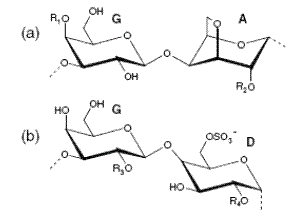Furcellaran is anionic partly sulphated polysaccharide which is classified together with carrageenan (E407).

The structure of furcellaran is similar to that of kappa carrageenan and has been described as a hybrid of kappa/beta carrageenans complex. The essential difference is that kappa carrageenan has one sulphate ester residue per two sugars, while furcellaran has one sulphate ester residue per three or four sugar residues.

Furcellaran is polysaccharide consisting of repeated disaccharide units of beta (R1 = R2 = H) and kappa carrageenan (R1 = SO3-, R2 = H). The structures of repeating disaccharide units of furcellaran and carrageenan are shown in figure 1.Figure 1. Furcellaran and carrageenan repeating disaccharide units

(a) kappa carrageenan (R1= SO3, R2= H), beta carrageenan (R1= R2=H),
furcellaran (R1= SO3, R2= H) (R1= R2= H), iota-carrageenan (R1= R2= SO3)

(b) lambda-carrageenan (R= R4= SO3)

Furcellaran is composed of D-galactose, 3,6-anhydro-D-galactose and D-galactose- 4-sulphate.